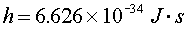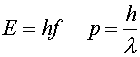What is quantum physics?

Quantum physics is necessary to understand the properties of solids, atoms, nuclei, subnuclear particles and light. In order to understand these natural phenomena, quantum principles have required fundamental changes in how humans view nature. To many philosophers (Einstein included), the conflict between the fundamental probabilistic features of quantum mechanics and older assumptions about determinism provided a cognitive shock that was even more unsettling that the revised views of space and time brought by special relativity.

The word quantum refers to discreteness, i.e., the existence of individual "lumps" as opposed to a continuum. In Newtonian physics, all quantities are allowed to be continuous. For instance, particles can have any momentum and light can have any frequency. A quantum is a discrete packet of energy, charge, or any other quantity. For instance, one might say that electric charge is quantized in units of e=1.602e-19 C. (Or in the case of quarks, units of e/3.)

In the next few pages, we will discuss the fact that all exchanges of energy come in discrete amounts. For instance, when light is absorbed by some material, the energy of the material does not rise continuously, but in discrete jumps. Each jump occurs when the material absorbs a single quantum of light. We will also learn that energy levels ("orbits") of an electron in an atom do not have a continuous range of possible energies, but instead that only discrete "orbits" are possible. This strange behavior is linked to the concept of wave-particle duality. We will see that particles can be described by wave functions that tell the probability of finding the particle.

A new fundamental constant must be introduced to account for all these new phenomena: Planck's constant. It is denoted by h.This constant relates wave-like quantities to particle-like ones. For instance, a particle's energy E is related to the frequency f of its wave function, and a particle's momentum p is related to the wavelength l of its wave function.Quantum physics index      examples        Lecture index